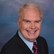# Math Solutions: Flow Velocity CalculationsBy Daniel Theobald, "Wastewater Dan"

Troubleshooting water/wastewater at your facility can sometimes be achieved by applying flow velocity math solution calculations.

Flow velocity is defined in fluid dynamics as the specific distance (feet, yards, or miles) fluids such as water and wastewater travel in an exact period of time including seconds, minutes, or hours.

In managing your water/wastewater, applying this tool can be very useful.

For example, it may be necessary to calculate the time that water flows from one location to another location, or there may be a need to monitor treatment effectiveness of water/wastewater flowing through single or multiple process units.

The basic flow velocity math solution calculation is expressed as x feet per second.

Often, it is more meaningful to customize the basic mathematical flow velocity calculation to express a specific distance and/or express a different time increment.

This tool for operators, represented through video tutorials, offers mathematical templates to customize flow velocity math word problem calculation solutions.

The tool also provides definitions, explanations, and alternative applications for the calculations beyond the specific examples presented. In addition to operators, it may also serve educators, engineers, regulators, and others.

A representative Flow Velocity Calculations math word problem and exact step-by-step solutions are presented  below.

After calculating flow velocity feet per second, how many hours will it take to flow wastewater by pumping from start to discharge with 1,000 feet from start in a 2” pipe at 25 gpm to a 55-gallon drum treatment tank, continuing with gravity-flow through the 55-gallon tank and down 167 yards from the tank in a 2” pipe to discharge?

Flow Velocity Calculation Solutions

Calculate flow velocity feet per second:

Calculate flow velocity feet per minute:

Calculate flow velocity minutes per 1,000 feet:

Calculate flow velocity hours per 1,000 feet:

Calculate detention time (D T) hours per 55 gallons:

Calculate flow velocity hours per 167 yards:

Calculate flow velocity hours from start to discharge:

Playlist of my 25-plus pump efficiency calculations

Playlist of all 170-plus Math Solutions

This is the Flow Velocity Calculations presentation in my series of “Math Solutions.” If you have specific wastewater math queries, please submit a question.